Courses

# Test: Operations On Sets

## 10 Questions MCQ Test Mathematics For JEE | Test: Operations On Sets

Description
This mock test of Test: Operations On Sets for JEE helps you for every JEE entrance exam. This contains 10 Multiple Choice Questions for JEE Test: Operations On Sets (mcq) to study with solutions a complete question bank. The solved questions answers in this Test: Operations On Sets quiz give you a good mix of easy questions and tough questions. JEE students definitely take this Test: Operations On Sets exercise for a better result in the exam. You can find other Test: Operations On Sets extra questions, long questions & short questions for JEE on EduRev as well by searching above.
QUESTION: 1

### Which of the following two sets are disjoint?

Solution:

{1,3,5}&{2,4,6} are disjoint sets.

Explanation:

In {1,3,5} & {1,3,6} 1 and 3 is the same numbers.

In {1,2,3} & {1,2,3} 1,2 and 3 is the same numbers.

In {1,3,5} & {2,3,4} 3 is the same number.

In {1,3,5} & {2,4,6} not any numbers are same.

QUESTION: 2

### The Shaded region in the following figure illustrates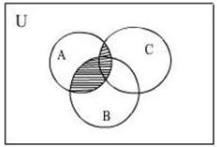Solution:

First which region is over which region Then We will see that A is on the B so A intersection B and after C is on the A so, A intersection C after that we have to take all intersection part so A intersection B is Union with A intersection C.

The shaded region represents (A ∩ B) ∪ (A ∩ C).

QUESTION: 3

### Shaded region in the following figure illustrates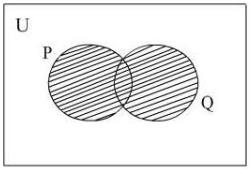Solution:

P U Q means P Union Q In set theory, the union (denoted by ∪) of a collection of sets is the set of all elements in the collection. It is one of the fundamental operations through which sets can be combined and related to each other.

QUESTION: 4

If the sets A and B are defined as= {(xy) : ex∈ R}; = {(xy) : x,∈ R}, then

Solution:

Since,ex andx do not meet for any∈ R∩ φ.

QUESTION: 5

The intersection of the sets {1, 2, 5} and {1, 2, 6} is the set ______

Solution:

The intersection of the sets A and B, is the set containing those elements that are in both A and B.

QUESTION: 6

From the following Venn diagram, A ∩ (B ∪ C) is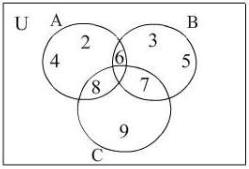Solution:

Explanation:- B = {3,5,6,7}   C = {7,8,9}

A = {2,4,6,8}

A⋂(B⋂C) = {6,8}

QUESTION: 7

If= {x is a multiple of 3} and= {x is a multiple of 5}, then A - B is

Solution: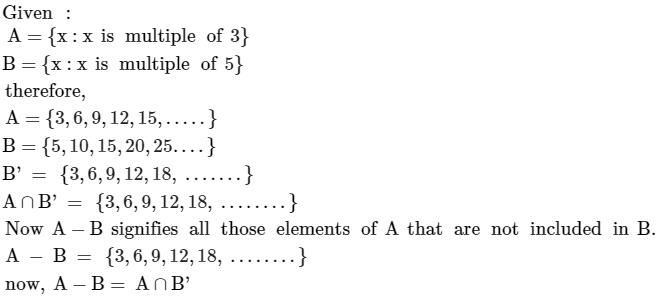QUESTION: 8

From the following venn diagram, A ∩ B is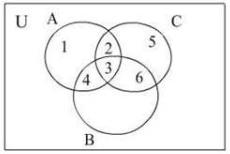Solution: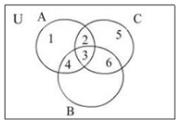A ∩ B = {4, 3}

QUESTION: 9

In probability, the event ‘A or B’ can be associated with set:

Solution:

Probability of event A or B

The probability that Events A and B both occur is the probability of the intersection of A and B. The probability of the intersection of Events A and B is denoted by P(A ∩ B). If Events A and B are mutually exclusive, P(A ∩ B) = 0. The probability that Events A or B occur is the probability of the union of A and B.

QUESTION: 10

If A and B are two given sets, then∩ (∩ B)' is equal to

Solution: